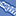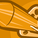# New to Qlik Sense

If you’re new to Qlik Sense, start with this Discussion Board and get up-to-speed quickly.

Announcements
Support Case Portal has moved to Qlik Community! Read the FAQs to start exploring Support resources.
cancel
Showing results for
Did you mean:Creator

## Set Analysis Expression

Hi All,

I am new in Qlik Sense, can you please help me out this expression what's I am doing mistake ?

sum of unit sold=sum({[Unit Sold]},Sales Data)

Labels (2)

• ### SaaS

1 Solution

Accepted SolutionsPartner

Hi Meera,

In script editior,

can you pls try this below expression

let vrevenue= 'Sum(Sales)*Sum(Stock)' // its working perfectly for me

if it not matching your answer then try separate variable in data load editor or front end  variable overview

let vsales= 'sum(Sales)';

let vstock= 'Sum(Stock)';

Let vrevenue= 'a*b';

i hope it will work

Regards,

Durga

3 RepliesPartner

Hi,

Set analysis mainly consist of set identifiers, set modifiers and set operators. if you want to understand the set analysis briefly means kindly visit the below link,

and you need a sum of unit sold then

=sum({\$}[Unit Sold])

here why you need an sales data or whether you filter out by Sales data

Regards,

DurgaCreator
Author

Thanks for your response I have sales data I want to calculate Revenue from two field one is unit sold and another price per unit.....

and I am doing this like

=Sum([Unit Sod])*Sum([Price per Unit])

and wants to assign variable like Let Revenue=Sum([Unit Sod])*Sum([Price per Unit])

but first issue that I am facing is - not able to find data load editor to use Let Command and  second my answer is showing wrong while calculating Revenue like the above expression.Partner

Hi Meera,

In script editior,

can you pls try this below expression

let vrevenue= 'Sum(Sales)*Sum(Stock)' // its working perfectly for me

if it not matching your answer then try separate variable in data load editor or front end  variable overview

let vsales= 'sum(Sales)';

let vstock= 'Sum(Stock)';

Let vrevenue= 'a*b';

i hope it will work

Regards,

DurgaTags
Community Browser